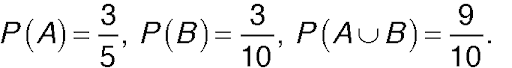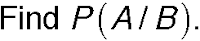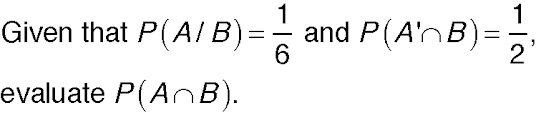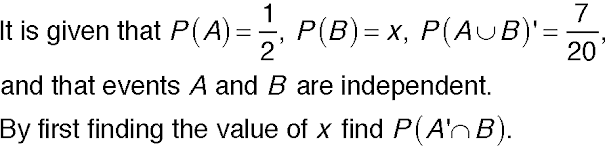IB Venn Diagrams and Probability
Questions 1 to 4. The diagram below shows the probability of a student picking Maths or Physics for a university course.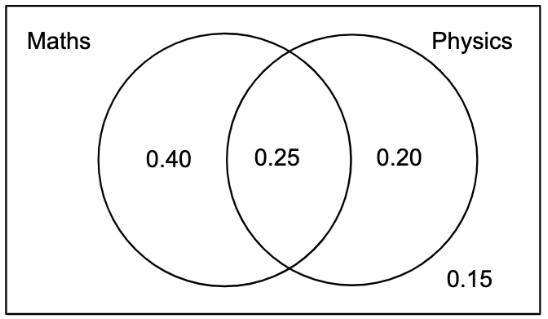1. Find the probability that a randomly chosen student is studying Maths.
1 point
2. Find the probability that a randomly chosen student is studying Physics only.
1 point
3. Find the probability that a randomly chosen student is studying Maths, given that they do Physics.
1 point
4. Find the probability that a randomly chosen student is studying Maths and Physics, given that they take at least one of these courses.
1 point
Question 5 to 8. The following shows some probabilities of events A and B occurring.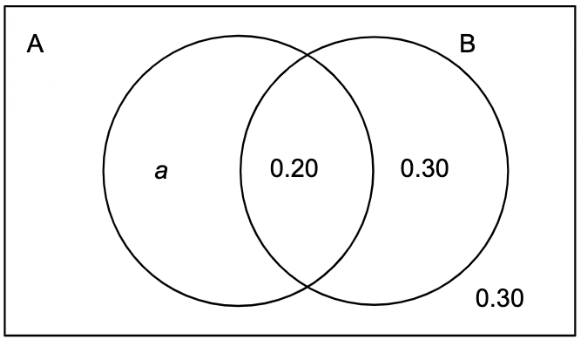5. Find the value of a.
1 point
6. Which of the following is true?
1 point
6. Which of the following is true?
1 point
7. Calculate P(A/B).
1 point
8. Calculate P(B/A).
1 point
Questions 9 to 11. In the following a, b, c, and d are all probabilities.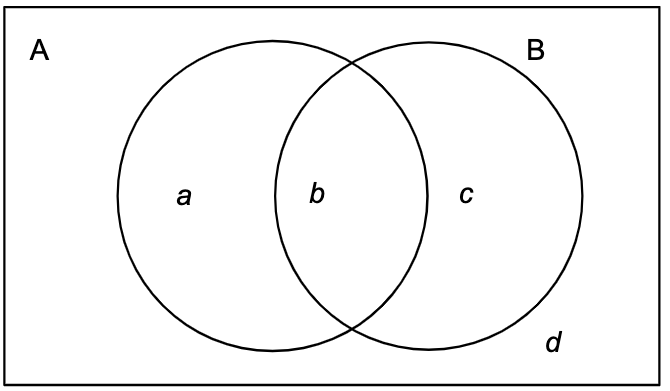1 point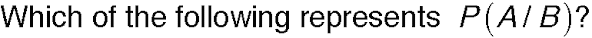1 point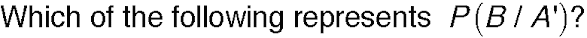11. Given events A and B are independent, which of the following is true?
1 point
Questions 12 to 13.